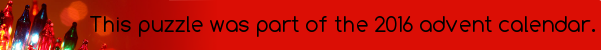mscroggs.co.uk
mscroggs.co.uksubscribe

## 12 DecemberHere is a list of facts about today's number:
• If a×b is a factor of it, with a and b both positive integers, then either a or b is one.
• The sum of its digits is 14.
• It is odd.
• The product of its digits is 36.
• It is a palindrome when written in base 9.
• It is smaller than yesterday's number.
• It is 4 more than a multiple of 5.
• It is two less than a prime number.
• It is the number of a bus stopping at Richmond station.
Tags: numbers

## Archive

Show me a random puzzle
▼ show ▼